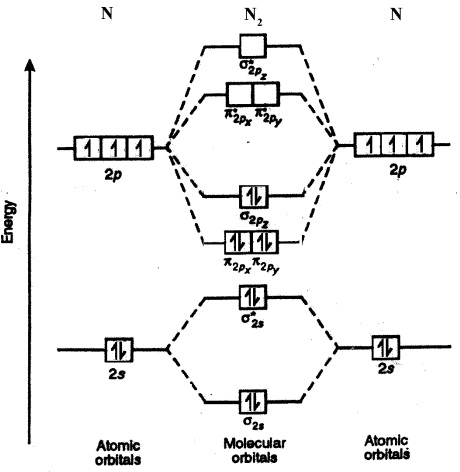# What type of bonding is in the sf(N_2) molecule ?

Jan 7, 2015

In the nitrogen molecule the atoms are held together by triple covalent bonds.

The electron structure of nitrogen is $1 {s}^{2} 2 {s}^{2} 2 {p}^{3}$'

There are therefore 5 valence electrons. These are able to form a triple bond with one non - bonding pair on each atom:The molecular orbital diagram is:The $2 {p}_{z}$ overlap "head on" to give a $\sigma$ bonding and a $\sigma \ast$ antibonding orbital.

The $2 {p}_{x}$ and $2 {p}_{y}$ are orthogonal (at right angles) and overlap to give two $\pi$ bonding orbitals and two $\pi \ast$ antibonding orbitals. Each pair ($2 {p}_{x}$ and $2 {p}_{y}$) overlaps like this:Here we can see that the number of bonding electrons ( ${N}_{b}$) is 8 and the number of antibonding electrons (${N}_{a}$) is 2.

The bond order $= \frac{\left({N}_{b} - {N}_{a}\right)}{2} = \frac{\left(8 - 2\right)}{2} = 3$